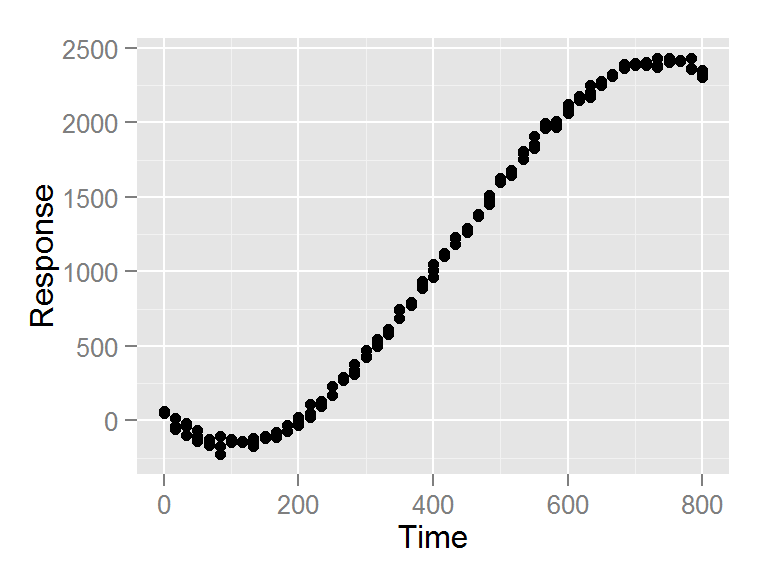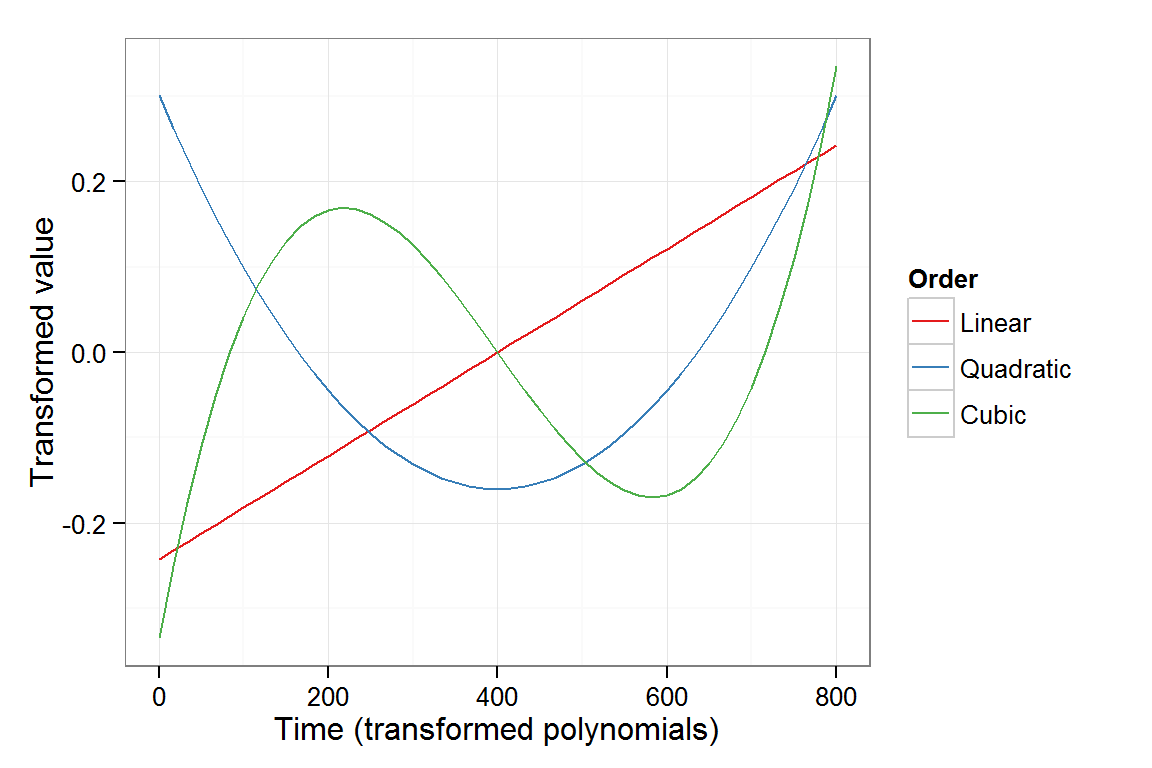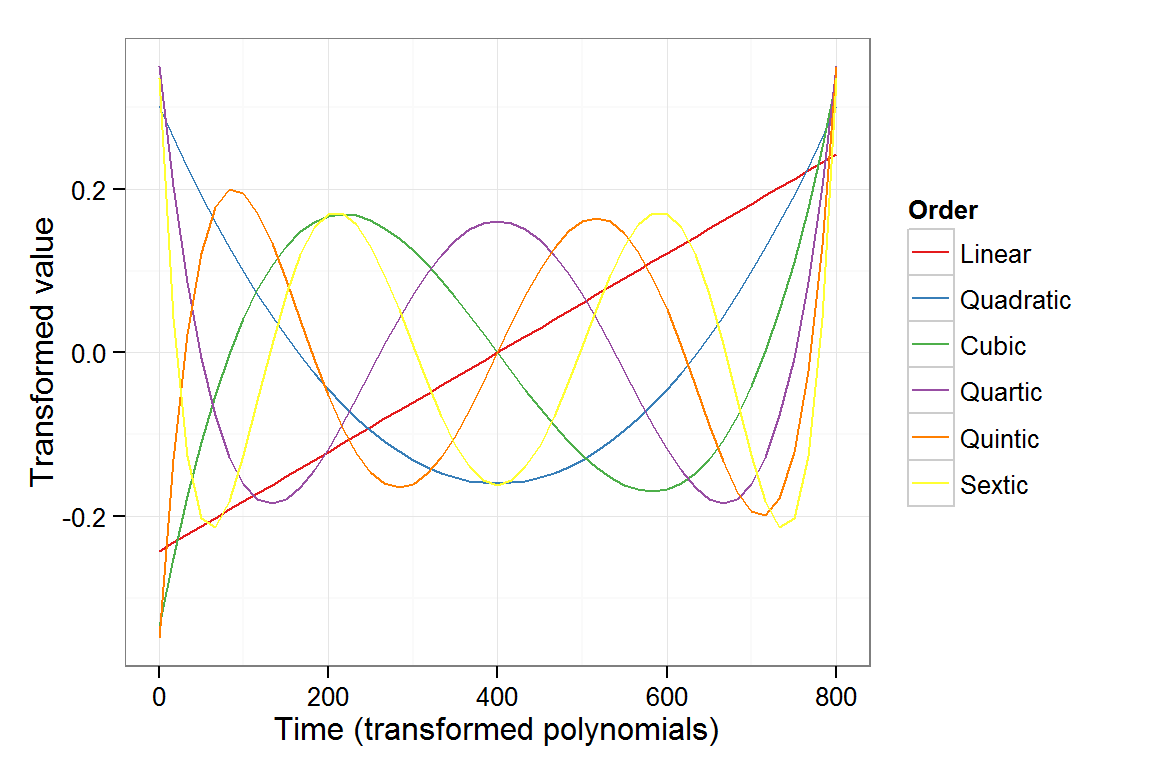Growth curve analysis can be described as fitting polynomial functions to your data.
When doing growth curve analysis in R, you have two options to implement polynomial curve fitting.
one is with the poly() function, which creates polynomial curves that span the range of your input, and take the canonical shape of their respective polynomial orders (e.g. linear, quadratic, cubic, etc.)

x <- 1:10
poly_x <- poly(x, degree = 3)
# see the vectors of polynomials
poly_x[,1:3]
##              1        2       3
##  [1,] -0.49543  0.52223 -0.4534
##  [2,] -0.38534  0.17408  0.1511
##  [3,] -0.27524 -0.08704  0.3779
##  [4,] -0.16514 -0.26112  0.3347
##  [5,] -0.05505 -0.34816  0.1296
##  [6,]  0.05505 -0.34816 -0.1296
##  [7,]  0.16514 -0.26112 -0.3347
##  [8,]  0.27524 -0.08704 -0.3779
##  [9,]  0.38534  0.17408 -0.1511
## [10,]  0.49543  0.52223  0.4534

Notice how the first column increases linearly, while the second column curves down and then up in a quadratic fashion, and the third column has two different deflections, like a cubic curve.

You can use this function directly in a formula within a modeling function like glm():

mod <- glm(output ~ poly(input, degree = 3), data=my.data)

This approach essentially substitutes all the vectors created above in place of the ‘input’ to the poly() function.
This method is described nicely on this R-Bloggers post.

Another way, which is explained eloquently in Dan Mirman’s book, is to explicitly code the levels of the polynomial in your data frame, and use those transformed polynomial values as predictors (rather than using your raw input values).

Dan’s website shows an excellent step-by-step approach to adding polynomials to your data frame. In this post, I demonstrate a function that you can use to shorten time time it takes you to implement polynomials in your data. It’s all based on Dan’s instructions, but with some different names and some extra sugar.
This updated function has been the result of a nice dialogue between Dan Mirman and myself. We’ve put some thoguht into accomodating different kinds of data, including missing and non-integer time bins. In this updated version, there’s less unnecessary addition of columns to the passed data frame. Additionally, the use of variable evaluation using [ rather than direct variable naming () eliminates the need to avoid specific predictor names. First let’s load up some packages library(ggplot2) library(reshape2) library(dplyr) Remove all objects and generate some data to have an object to work with rm(list=ls()) times <- seq(0, 800, 16.66) my_data <- data.frame(Time = rep(times, 3)) %>% mutate(Response = 1000 + # linear component (((Time - 400)*6) ^ 1) + # quadratic component (((Time - 400)*-0.0350) ^ 2) + # cubic component (((Time - 400)*-0.0270) ^ 3)) %>% mutate(Response = Response + rnorm(n = 3*length(times), mean = 0, sd = 25)) see the data ggplot(my_data, aes(x=Time, y=Response))+ geom_point()Side note: when creating a function like this, I usually start out with some global variable declarations so that I can run the function line-by-line and see the output as it runs along. At this point the function is complete, so this can be ignored, but in the spirit of being honest, this was an important part of the story. # dummy variables to debug within the function # df <- my_data # predictor <- "time" # poly.order <- 3 # orthogonal <- TRUE ### Here’s the function! df is your data frame, predictor is your continuous predictor variable (usually time) poly.order is the degree of polynomials that you wish to use. (i.e. 2 for quadratic, 3 for cubic, etc) orthogonal is TRUE by default, but you can set it to FALSE to use raw polynomials. If you’re reading this page, you are probably interested in orthogonal polynomials, so that you can measure separate independent linear, quadratic, cubic, etc. terms. draw.poly as TRUE will display a colored ggplot that illustrates the polynomial shapes. #' Build polynomial predictor variables #' #' Takes a data frame, name of predictor variable, and polynomial order. Creates polynomial-transformed predictor variables, adds them to the data frame and returns the result. Original data frame is unchanged, remember to assign the result. #' #' @param df data frame, should not contain any variables called "predictor" #' @param predictor string name of predictor variable #' @param poly.order integer order of polynomial to be created #' @param orthogonal logical value indicating whether polynomial should be orthogonal (default) or natural (aka raw) #' @param draw.poly logical value indicating whether to create a graph showing tranformed polynomial predictor values, defaults to TRUE #' @return Returns a data frame containing the original data and at least two new columns: "predictor".Index, and a column for each order of the polynomial-transformed predictor #' @examples #' WordLearnEx.gca <- code.poly(df=WordLearnEx, predictor="Block", poly.order=2) #' Az.gca <- code.poly(df=Az, predictor="Time", poly.order=2, orthogonal=FALSE) #' @section Contributors: #' Originally written by Matt Winn \url{http://www.mattwinn.com/tools/R_add_polynomials_to_df.html} and revised by Dan Mirman to handle various corner cases. code.poly <- function(df=NULL, predictor=NULL, poly.order=NULL, orthogonal=TRUE, draw.poly=TRUE){ require(reshape2) require(ggplot2) # Codes raw or orthogonal polynomial transformations of a predictor variable # be sure to not have an actual variable named "predictor" in your data.frame # ultimately adds 2 or more columns, including: # (predictor).Index, and a column for each order of the polynomials # # Written by Matt Winn: http://www.mattwinn.com/tools/R_add_polynomials_to_df.html # Revised by Dan Mirman (11/3/2014): # - call to poly now uses predictor.index and 1:max instead of unique() to deal with out-of-order time bins # - combined indexing and alignment with original data, mainly to avoid problems when poly.order==1 # Revised by Matt Winn (2/12/2015) # - uses [ instead of  to use variable name to extract column
#   - computes polynomial on unique(sort(predictor.vector))
#       rather than 1:max(predictor.vector)
#       to accomodate non-integer predictor (e.g. time) levels
#   - Accomodates missing/unevenly-spaced time bins
#       by indexing each sorted unique time bin and using the index to extract
#       the polynomial value

#===========================================================#
# convert choice for orthogonal into choice for raw
raw <- (orthogonal-1)^2

# make sure that the declared predictor is actually present in the data.frame
if (!predictor %in% names(df)){
warning(paste0(predictor, " is not a variable in your data frame. Check spelling and try again"))
}

# Extract the vector to be used as the predictor
predictor.vector <- df[,which(colnames(df)==predictor)]

# create index of predictor (e.g. numbered time bins)
# the index of the time bin will be used later as an index to call the time sample
predictor.indices <- as.numeric(as.factor(predictor.vector))

dftemp.predictor.index <- predictor.indices #create x-order order polys (orthogonal if not raw) predictor.polynomial <- poly(x = unique(sort(predictor.vector)), degree = poly.order, raw=raw) # use predictor index as index to align # polynomial-transformed predictor values with original dataset # (as many as called for by the polynomial order) df[, paste("poly", 1:poly.order, sep="")] <- predictor.polynomial[predictor.indices, 1:poly.order] # draw a plot of the polynomial transformations, if desired if (draw.poly == TRUE){ # extract the polynomials from the df df.poly <- unique(df[c(predictor, paste("poly", 1:poly.order, sep=""))]) # melt from wide to long format df.poly.melt <- melt(df.poly, id.vars=predictor) # Make level names intuitive # don't bother with anything above 6th order. levels(df.poly.meltvariable)[levels(df.poly.melt$variable)=="poly1"] <- "Linear" levels(df.poly.melt$variable)[levels(df.poly.melt$variable)=="poly2"] <- "Quadratic" levels(df.poly.melt$variable)[levels(df.poly.melt$variable)=="poly3"] <- "Cubic" levels(df.poly.melt$variable)[levels(df.poly.melt$variable)=="poly4"] <- "Quartic" levels(df.poly.melt$variable)[levels(df.poly.melt$variable)=="poly5"] <- "Quintic" levels(df.poly.melt$variable)[levels(df.poly.melt\$variable)=="poly6"] <- "Sextic"

# change some column names for the output
colnames(df.poly.melt)[colnames(df.poly.melt) == "variable"] <- "Order"

poly.plot <- ggplot(df.poly.melt, aes(y=value, color=Order))+
aes_string(x=predictor)+
geom_line()+
xlab(paste0(predictor, " (transformed polynomials)"))+
ylab("Transformed value")+
scale_color_brewer(palette="Set1")+
theme_bw()

print(poly.plot)
}

# restore correct column names
colnames(df)[colnames(df) == "temp.predictor.index"] <- paste0(predictor,".Index")
return(df)
}

## Here’s an example of how the function is called

using the data that we created at the beginning of the script.

data.gca <- code.poly(df=my_data, predictor="Time", poly.order=3, orthogonal=TRUE, draw.poly=TRUE)Now view data.gca to see how the time index and polynomials have been added to the df.

head(data.gca)
##    Time Response Time.Index   poly1  poly2     poly3
## 1  0.00    51.91          1 -0.2424 0.3005 -0.334370
## 2 16.66   -36.50          2 -0.2323 0.2629 -0.250778
## 3 33.32   -34.47          3 -0.2222 0.2269 -0.176078
## 4 49.98  -103.61          4 -0.2121 0.1926 -0.109884
## 5 66.64  -127.17          5 -0.2020 0.1598 -0.051810
## 6 83.30  -172.46          6 -0.1919 0.1287 -0.001469

And a simple model

library(lme4)
mod <- lm(Response ~ poly1 + poly2 + poly3, data=data.gca)
summary(mod)
##
## Call:
## lm(formula = Response ~ poly1 + poly2 + poly3, data = data.gca)
##
## Residuals:
##    Min     1Q Median     3Q    Max
## -76.17 -19.29   1.45  17.81  53.89
##
## Coefficients:
##             Estimate Std. Error t value Pr(>|t|)
## (Intercept)  1069.99       2.16   495.8   <2e-16 ***
## poly1        6667.02      15.11   441.3   <2e-16 ***
## poly2         431.63      15.11    28.6   <2e-16 ***
## poly3       -1408.96      15.11   -93.3   <2e-16 ***
## ---
## Signif. codes:  0 '***' 0.001 '**' 0.01 '*' 0.05 '.' 0.1 ' ' 1
##
## Residual standard error: 26.2 on 143 degrees of freedom
## Multiple R-squared:  0.999,  Adjusted R-squared:  0.999
## F-statistic: 6.81e+04 on 3 and 143 DF,  p-value: <2e-16

Now instead of repeating the multi-step pocess of adding polynomials, you can run a one-line function and make life easier!

## Now, just for fun…

What does it look like to have a 6th-order polynomial?
lets find out…

data.gca6 <- code.poly(df=my_data, predictor="Time",poly.order=6, orthogonal=TRUE, draw.poly=TRUE)Whoa.

This is an R Markdown document. Markdown is a simple formatting syntax for authoring HTML, PDF, and MS Word documents. For more details on using R Markdown see http://rmarkdown.rstudio.com.